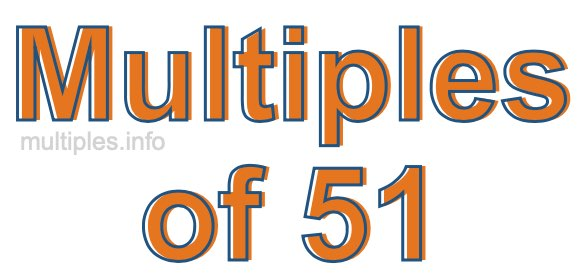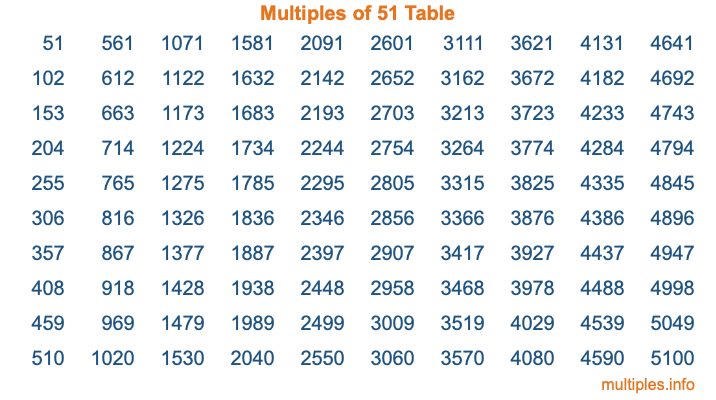Multiples of 51Welcome to the Multiples of 51 page. Here we will first teach you everything you will ever need to know about the multiples of 51, and then give you a study guide summary of everything we taught you to make sure you remember it all. Use this page to look up facts and learn information about the multiples of 51. This page will make you a multiples of fifty-one expert!

Definition of Multiples of 51
Multiples of 51 are all the numbers that when divided by 51 equal an integer. Each of the multiples of 51 are called a multiple. A multiple of 51 is created by multiplying 51 by an integer.

Therefore, to create a list of multiples of 51, you start with 1 multiplied by 51, then 2 multiplied by 51, then 3 multiplied by 51, and so on for as long as you want. Thus, the list of the first five multiples of 51 is 51, 102, 153, 204, and 255. To see a larger list of multiples of 51, see the printable image of Multiples of 51 further down on this page. We also have a category where you can choose any nth multiple of 51.

Multiples of 51 Checker
The Multiples of 51 Checker below checks to see if any number of your choice is a multiple of 51. In other words, it checks to see if there is any number (integer) that when multiplied by 51 will equal your number. To do that, we divide your number by 51. If the the quotient is an integer, then your number is a multiple of 51.

Is  a multiple of 51?

Least Common Multiple of 51 and ...
A Least Common Multiple (LCM) is the lowest multiple that two or more numbers have in common. This is also called the smallest common multiple or lowest common multiple and is useful to know when you are adding our subtracting fractions. Enter one or more numbers below (51 is already entered) to find the LCM.

Check out our LCM Calculator if you need more details about the Least Common Multiple or if you need the LCM for different numbers for adding and subtraction fractions.

nth Multiple of 51
As we stated above, 51 is the first multiple of 51, 102 is the second multiple of 51, 153 is the third multiple of 51, and so on. Enter a number below to find the nth multiple of 51.

th multiple of 51

Multiples of 51 vs Factors of 51
51 is a multiple of 51 and a factor of 51, but that is where the similarities end. All postive multiples of 51 are 51 or greater than 51. All positive factors of 51 are 51 or less than 51.

Below is the beginning list of multiples of 51 and the factors of 51 so you can compare:

Multiples of 51: 51, 102, 153, 204, 255, etc.

Factors of 51: 1, 3, 17, 51

As you can see, the multiples of 51 are all the numbers that you can divide by 51 to get a whole number. The factors of 51, on the other hand, are all the whole numbers that you can multiply by another whole number to get 51.

It's also interesting to note that if a number (x) is a factor of 51, then 51 will also be a multiple of that number (x).

Multiples of 51 vs Divisors of 51
The divisors of 51 are all the integers that 51 can be divided by evenly. Below is a list of the divisors of 51.

Divisors of 51: 1, 3, 17, 51

The interesting thing to note here is that if you take any multiple of 51 and divide it by a divisor of 51, you will see that the quotient is an integer.

Multiples of 51 Table
Below is an image of the first 100 multiples of 51 in a table. The table is in chronological order, column by column. The first column has the first ten multiples of 51, the second column has the next ten multiples of 51, and so on.The Multiples of 51 Table is also referred to as the 51 Times Table or Times Table of 51. You are welcome to print out our table for your studies.

Negative Multiples of 51
Although not often discussed or needed in math, it is worth mentioning that you can make a list of negative multiples of 51 by multiplying 51 by -1, then by -2, then by -3, and so on, to get the following list of negative multiples of 51:

-51, -102, -153, -204, -255, etc.

Multiples of 51 Summary
Below is a summary of important Multiples of 51 facts that we have discussed on this page. To retain the knowledge on this page, we recommend that you read through the summary and explain to yourself or a study partner why they hold true.

There are an infinite number of multiples of 51.

A multiple of 51 divided by 51 will equal a whole number.

51 divided by a factor of 51 equals a divisor of 51.

The nth multiple of 51 is n times 51.

The largest factor of 51 is equal to the first positive multiple of 51.

51 is a multiple of every factor of 51.

51 is a multiple of 51.

A multiple of 51 divided by a divisor of 51 equals an integer.

51 divided by a divisor of 51 equals a factor of 51.

Any integer times 51 will equal a multiple of 51.

Multiples of a Number
Here you can get the multiples of another number, all with the same attention to detail as we did for multiples of 51 on this page.

Multiples of
Multiples of 52
Did you find our page about multiples of fifty-one educational? Do you want more knowledge? Check out the multiples of the next number on our list!